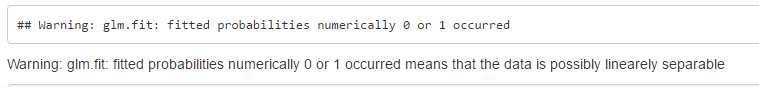## Regression Analysis

Hello

1) In logistic regression, if confidence interval includes the value 0 then what will be the conclusion on significance of the variable.

2) How to decide whether the data is Linear or Linearly Separable?.

Thanks

1 ACCEPTED SOLUTION

Accepted Solutions

## Re: Regression Analysis

@KafeelBasha wrote:

1) Is there any specific reason behind saying a variable is insignificant if the confidence interval includes 0.

2) I came across below warning message while going through an example of logistic regression in R

1) Because if it includes 0 in the interval that means a valid value is 0, which is no difference, ie not significant difference. I would suggest re-reading how confidence interval, p-values and hypothesis testing all relate.

2) Ask R questions in an R forum, ask SAS question in a SAS forum. But it means that some variables in your data are essentially the same. For example, a very basic examples is if you had two variables that are X=1 if A is present, 0 otherwise and Y=1 if A is not present, otherwise Y=0. They're the same.

4 REPLIES 4Ksharp
Super User

## Re: Regression Analysis

1) it is not significant.

2)don't understand your question. You mean linear or nonlinear relationship between X and Y ?

Check EFFECT statement.

Or you should check Q-Q plot .

## Re: Regression Analysis

1) Is there any specific reason behind saying a variable is insignificant if the confidence interval includes 0.

2) I came across below warning message while going through an example of logistic regression in Rhttp://michael.hahsler.net/SMU/EMIS7332/R/logistic_regression.html

When can we say the data is linear or Linearly Separable?

## Re: Regression Analysis

@KafeelBasha wrote:

1) Is there any specific reason behind saying a variable is insignificant if the confidence interval includes 0.

2) I came across below warning message while going through an example of logistic regression in R

1) Because if it includes 0 in the interval that means a valid value is 0, which is no difference, ie not significant difference. I would suggest re-reading how confidence interval, p-values and hypothesis testing all relate.

2) Ask R questions in an R forum, ask SAS question in a SAS forum. But it means that some variables in your data are essentially the same. For example, a very basic examples is if you had two variables that are X=1 if A is present, 0 otherwise and Y=1 if A is not present, otherwise Y=0. They're the same.Ksharp
Super User

## Re: Regression Analysis

```H0: b=0 , so if b -> [-x,x ] then it can not indicate if b=0 or not .

"probability numerically 0 or 1"
It is more like SAS 's message "can't separate likelihood".

Maybe your CLASS variable have some level which is dropout.
E.X.
sex=F appeared only once .